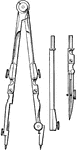Compasses

"The compasses, next to the T square and triangles, are used more than any other instrument. A pencil…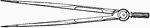Compasses

"The compasses, next to the T square and triangles, are used more than any other instrument. A pencil…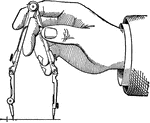Compasses

"The compasses, next to the T square and triangles, are used more than any other instrument. A pencil…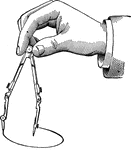Compasses

"The compasses, next to the T square and triangles, are used more than any other instrument. A pencil…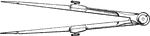Compasses

"The compasses, next to the T square and triangles, are used more than any other instrument. A pencil…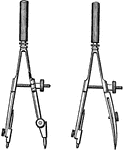Compasses

"The compasses, next to the T square and triangles, are used more than any other instrument. A pencil…Architects Drafting Square

A T-square is a technical drawing instrument, primarily a guide for drawing horizontal lines on a drafting…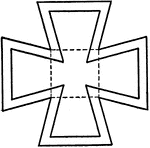Drawing Maltese Cross using T-square, Spacers, and Triangles

"Draw three-inch square and one-inch square. From the corners of inner square draw lines to outer square…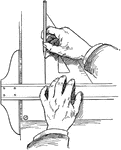Drawing Vertical Lines Using a T-square and a Triangle

When drawing vertical lines, the blade of the T-square is held with the thumb while the fingers adjusting…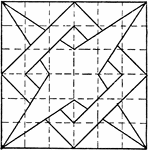Constructing 4 Point Star Overlapping Box Pattern using T Square and Triangle

A problem exercise to construct a star overlapping square using a triangle and T square. The image is…Constructing Star and Box Overlap Pattern using T Square and Triangle

The construction of a star with square overlapping each other using a triangle and T square. This image…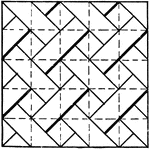Drawing Exercise for Drawing Diagonal Line Pattern using T Square and Triangle

A diagonal line pattern drawing exercise in for T square and triangle. The image is constructed by dividing…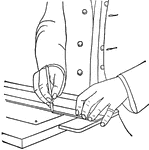T Square

A person using a T square to draw straight lines.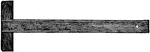T-square

A T-square is used to aid drawing straight and parallel lines. It consist of a thin straightedge, the…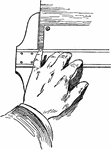T-square Holding Position Using Fingers on Blade

T-squares is used on the left side of the board for righted handed people, and right side of the board…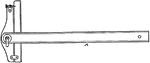An adjustable T-square to create straight lines at different angles with a ruler attached to the head.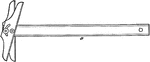An adjustable T-square to create straight lines at different angles.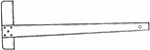English T-square

An English T-square for drawing straight lines. The narrowing of the bottom blade makes the T-square…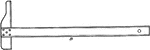Long Fixed Head T-square

A long fixed head T-square for drawing straight lines. The head shape of the T-square allows for drawing…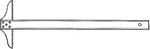Regular Fixed Head T-square

A regular fixed head T-square for drawing straight lines. The blade must be perfectly straight in order…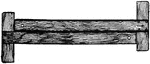Testing The Edge of T-squares

To test the straightness of the edge two T-squares may be placed together.